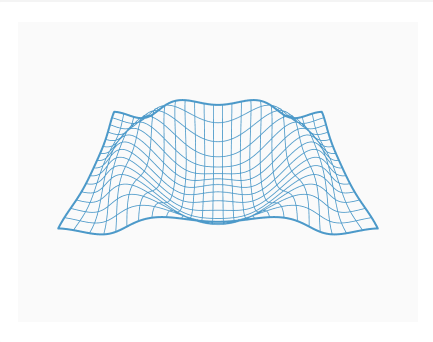#### Howdy, Stranger!

We are about to switch to a new forum software. Until then we have removed the registration on this forum.

# "Size() cannot be used here" Error for "size (800, 600, P3D)"

edited October 2016

I am new to Processing. I picked up the book Processing: A Programming Handbook for Visual Designers and Artists so started going through that. I have been trying out code that I have come across from others and had an error, that I wanted to ask the forum for help. I am running Processing 3.2.1 on Windows 10 but getting an error on the below code "size (800, 600, P3D)" the window at bottom says size() cannot be user here. If someone can kindly review the below code and let me know what needs to be changed, I would greatly appreciate it. Thanks https://dribbble.com/shots/1754428-WaveCode below

``````            // by d whyte

int[][] result;
float t;

float ease(float p) {
return 3*p*p - 2*p*p*p;
}

float ease(float p, float g) {
if (p < 0.5)
return 0.5 * pow(2*p, g);
else
return 1 - 0.5 * pow(2*(1 - p), g);
}

float mn = .5*sqrt(3);

void setup() {
setup_();
result = new int[width*height];
}

void draw() {

if (!recording) {
t = mouseX*1.0/width;
draw_();
} else {
for (int i=0; i<width*height; i++)
for (int a=0; a<3; a++)
result[i][a] = 0;

for (int sa=0; sa<samplesPerFrame; sa++) {
t = map(frameCount-1 + sa*shutterAngle/samplesPerFrame, 0, numFrames, 0, 1);
draw_();
for (int i=0; i<pixels.length; i++) {
result[i] += pixels[i] >> 16 & 0xff;
result[i] += pixels[i] >> 8 & 0xff;
result[i] += pixels[i] & 0xff;
}
}

for (int i=0; i<pixels.length; i++)
pixels[i] = 0xff << 24 |
int(result[i]*1.0/samplesPerFrame) << 16 |
int(result[i]*1.0/samplesPerFrame) << 8 |
int(result[i]*1.0/samplesPerFrame);
updatePixels();

saveFrame("f###.gif");
if (frameCount==numFrames)
exit();
}
}

//////////////////////////////////////////////////////////////////////////////

int samplesPerFrame = 16;
int numFrames = 96;
float shutterAngle = .5;

boolean recording = true;

void setup_() {
size(800, 600, P3D);
smooth(8);
rectMode(CENTER);
stroke(70,150,200);
noFill();
strokeWeight(2);
}

float x, y, z, tt;
int N = 24, M = 16;
float l = 500, w = 320;
int n = 120;
float h = 120;
float q = 0.00011, ll = .000035;

void draw_() {
background(250);
pushMatrix();
translate(width/2, height/2 - 25);
rotateX(PI*.24);
for (int i=0; i<N; i++) {
x = map(i, 0, N-1, -l/2, l/2);
strokeWeight(1.6);
if(i==0 || i==N-1)
strokeWeight(4);
beginShape();
for (int j=0; j<n; j++) {
y = map(j, 0, n-1, -w/2-1, w/2+1);
z = h*sin(TWO_PI*t - q*(x*x + y*y))*exp(-ll*(x*x + y*y));
vertex(x, y, z);
}
endShape();
}
for (int i=0; i<M; i++) {
y = map(i, 0, M-1, -w/2, w/2);
strokeWeight(1.6);
if(i==0 || i==M-1)
strokeWeight(4);
beginShape();
for (int j=0; j<n; j++) {
x = map(j, 0, n-1, -l/2-1, l/2+1);
z = h*sin(TWO_PI*t - q*(x*x + y*y))*exp(-ll*(x*x + y*y));
vertex(x, y, z);
}
endShape();
}
popMatrix();
}
``````Tagged:

• I wanted to ask if others can past the corrected text back for me to run. Also, I wanted to ask how to format the code using Markdown formatting. When I pasted the code, it did not keep the formatting I had.

• Just what there error message says. If you look up the Processing reference entry for size():

In a program that has the setup() function, the size() function must be the first line of code inside setup().

`size()` must be called in `setup()`, but currently, you are having the standard `setup()` call your own custom function `setup_()` -- I'm not sure why -- and then calling `size` there. Don't do that. Move it. Does the error go away?

• ... the size() function MUST be the first line of code inside setup().

I only send folks to Processing API's online reference due to laziness. I-)
But unfortunately we should NEVER "believe" everything said there at all! [-(
There are lotsa of superstitious statements and even serious omissions there! :^o

In this particular case, size() can be placed anywhere within setup().
The abusive use of MUST verbs throughout the online reference is laughable to say the least! :O)
Although it's indeed recommended, rather than obligated, to place size() as the 1st statement. :-j

Now the actual "bug" in your code is that size() isn't located within setup(), rather it's called from it! :-B
Since Processing 3, all size(), fullScreen(), pixelDensity(), smooth(), noSmooth() functions have to be called from settings().

When using the PDE (Processing's IDE), its pre-processor automatically searches the setup() callback for those aforementioned functions. Each 1 found is then moved into settings()! @-)
We can verify that by CTRL+SHIFT+E to export our sketch; and then check its generated ".java" file! :ar!

• edited October 2016

Thank you for your guidance. Is it possible to request to drop the completed corrected code this here for me to compare and run? Again, completely new to Processing and still don't fully follow the corrections? Thank you in advance. Sorry for the for the freshman question.

• Regardless if you understood the tech reasons or not, the easiest fix for your case is to simply move everything in setup_() into setup()! >-)

• Thank you. Is it possible to drop that correction here. Still don't fully follow what you mean.

``````void setup() {
setup_();
result = new int[width*height];
}

void setup_() {
size(800, 600, P3D);
smooth(8);
rectMode(CENTER);
stroke(70,150,200);
noFill();
strokeWeight(2);
}
``````

are merged as 1:

``````void setup() {
size(800, 600, P3D);
smooth(8);
rectMode(CENTER);
stroke(70,150,200);
noFill();
strokeWeight(2);
result = new int[width*height];
}
``````